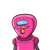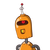# A rare whale fossil was uncovered under layers of sediment. When the excavator took measurements, she found the tail to be as long

A rare whale fossil was uncovered under layers of sediment. When the excavator took measurements, she found the tail to be as long as its head plus a quarter of the length of the body. When the archaeologist came in, he measured the length of the body. He found it to be ¾ of the total length. They made arguments about how such a fish would swim in the water. To make proper predictions they measured the head size, and found it to be 4 inches long. What is the total length of this whale?
A)128 B)99 C)136 D)142
SPAMS ARE STRICTLY BLOCKED​

### 2 thoughts on “A rare whale fossil was uncovered under layers of sediment. When the excavator took measurements, she found the tail to be as long”

1.Step-by-step explanation:

[tex]\huge\tt\fbox\purple{Given:-}[/tex]

=> The tail is to be as long as it’s head plus a quarter of the length of the body.

=> Length of body be ¾ of the length.

=> Head is 4 inches long.

[tex]\huge\tt\fbox\purple{To Find:- }[/tex]

=> The total length of whale.

[tex]\huge\tt\fbox\purple{Solution:-

}[/tex]

=> Body = x inch

=> Tail = Head + 1/4x

=> Tail = 4 + x/4 inches.

Total length = Head + Body + Tail

=> 4+x+4+x/4

=> 8 + 5x/4

Body = 3/4T Total length

x = 3/4 (8 + 5x/4)

4x = 3 × 32 + 5x/4

16x = 96 + 15x

x = 96

Total length = 8 + 5 × 96/4

=> 8 + 5 × 24

=> 8 + 120

=> 128

=> Body = 96 inches

=> Tail = 28 inches

Therefore, The total length of the whale is 128inches.

2.